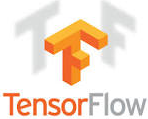As you know now technology moving towards Machine learning/Deep learning so if you are making career in it then your future is bright, you will got very high package :). Here we come with Google TensorFlow interview questions, our previous article "100 Machine learning Interview Q-A" was become very famous so must go through it.
Future of TensorFlow: TensorFlow future bright , TensorFlow is growing fast, especially when someone like Google create such thing, its likely to be big, one of the reason is because they are using in their own products which encourages others to use it also.What is TensorFlow?
TensorFlow is a Python library for fast numerical computing created and released by Google.
It is a foundation library that can be used to create Deep Learning models directly or by using wrapper libraries that simplify the process built on top of TensorFlow.
Unlike other numerical libraries intended for use in Deep Learning like Theano, TensorFlow was designed for use both in research and development and in production systems.
It can run on single CPU systems, GPUs as well as mobile devices and large scale distributed systems of hundreds of machines. Answer credit goest to : Jason Brownlee

What is necessary to evaluate any formulas in Tensorflow?
with tf.Session() as sess:

How do you sum the whole array into one number in tensorflow?
tf.reduce_sum(array) or tf.reduce_sum(x, [0, 1])

What programming language is it for?
The API is nominally for the Python programming language, although there is access to the underlying C++ API.

What do you understand by Tensor and Flow in case of Tensorflow?
Tensor:
• Multi dimensional array - e.g., scalar, vector, matrix, cube
Flow:
• A graph that defines operations like + to do with data (tensors).
• A lot like numpy
• Fast Math with tensors
What is nodes?
Nodes perform computation and have zero or more inputs and outputs. Data that moves between nodes are known as tensors, which are multi-dimensional arrays of real values.

What is edges?
The graph defines the flow of data, branching, looping and updates to state. Special edges can be used to synchronize behavior within the graph, for example waiting for computation on a number of inputs to complete.

What is the purpose of tf.Session?
It provides a class for running Tensorflow objects. It encapsulates the environment in which Operation objects are executed and Tensor objects are evaluated.
usage:
with tf.Session as sess:
(context manager)
or
sess = tf.Session()
which then requires
sess.run(...)
sess.close()

What happens when you create a variable?
You pass a tensor into the Variable() constructor. You must specify the shape of the tensor which becomes the shape of the variable. Variables generally have fixed shape.

When you will use tf.get_variable()?
Sometimes you have large sets of variables in complex models that you want to all initialize in the same place.

What does tf.get_variable() do?
It creates or returns a variable with a given name instead of a direct call to tf.Variable(). It uses an initializer instead of calling tf.Variable directly

What does the softmax cross entropy function do?
Measures the probability error in discrete classification tasks in which the classes are mutually exclusive (each entry is in exactly one class).

1.2.3.